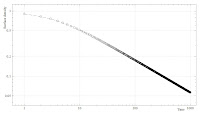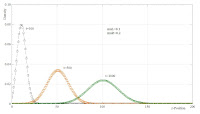## Wednesday, April 29, 2009

### Cell invasion (1) : Biased diffusion model

Consider living cells that are initially located at the surface (x-,y-plane) of a half-space of dense medium (positive z-axis of coord. system pointing into the medium, z=0 at the surface). As shown in the figure below, the cells can invade into the volume of the medium, migrate there and possibly return to the surface.• As the simplest possible model, we assume that the cells perform a (biased) random walk with statistically independent spatial components (dx,dy,dz) of the displacements.
• We are only interested in the temporal evolution of the probability density function P(z,t) of the cell's z-position. Since the 3 coordinates are independent random processes, we consider the whole problem as a 1D-diffusion problem with special boundary conditions and with possible trends (drift terms).
• The trends could, for example, describe the effect of a chemo-attractant in the medium, creating a bias of diffusion into the positive z-direction (later called the "right" direction). A chemo-repellant would cause a drift to the "left".

The corresponding Fokker-Planck equation for the temporal evolution of P(z,t) could be solved analytically. Instead we discretize the problem spatially (z-segments in the figure) and temporally and integrate the resulting master equation numerically. The surface is described by the special segment Z=0.

For given resolutions (dz, dt), we define 4 dimensionless parameters:

• muR = fraction of particles in segment Z that move to the segment Z+1 to the right within one time step.
• muL=fraction moving to the left.
• muV=fraction of particles on the surface (Z=0) that move into the volume.
• muS=fraction of particles in volume segment (Z=1) that move to the surface.
Let
P_z^{(t)}
denote the normalized probability density (PDF) of particles in segment z at time step t.

The initial condition is
P_z^{(0)}=\delta_{z0}.

The master equation reads for the surface segment
P_0^{(t+1)}=(1-\mu_V) P_0^{(t)} + \mu_S P_1^{(t)},

for the volume segment Z=1
P_1^{(t+1)}=(1-\mu_S-\mu_R) P_1^{(t)} + \mu_V P_0^{(t)} + \mu_L P_2^{(t)},

and for all other segments Z>1
P_z^{(t+1)}=(1-\mu_L-\mu_R) P_z^{(t)} + \mu_R P_{z-1}^{(t)} + \mu_L P_{z+1}^{(t)}.

First, we shall treat the passage of the cell through the s pecial boundary between the last segment Z=1 and the surface segment Z=0 in the same way as for all other segments:
\mu_V=\mu_R \; , \; \mu_S=\mu_L.

---------------------------------------------------------

Simulation results for symmetric conditions:
\mu_R=\mu_L=0.1Here, the density profiles are approximately Half-Gaussians, peaked at the surface, with temporally increasing variance. The surface density decays at long times according to a t^(-1/2) powerlaw.

---------------------------------------------------------

Simulation results with "chemo-attractant":
\mu_L=0.1 \;,\; \mu_R=0.2Here, the peak of the Gaussian profiles is drifting to the right. The surface density decays exponentially.

---------------------------------------------------------

Simulation results with "chemo-repellant":
\mu_L=0.2 \;,\; \mu_R=0.1Here, the profiles quickly approach a stationary, exponential distribution. The surface density falls to a stationary, finite value.

---------------------------------------------------------

We will next compare those simulations with measured cell invasion data.